# Учебный центр

## Handling Complex-Valued Numbers and Problems in the Frequency Domain

Here, we address how COMSOL Multiphysics® handles complex-valued numbers and problems in the frequency domain. We discuss when you should use the root-mean-square (RMS), peak, or instantaneous value to specify sources in models being solved in the frequency domain, such as for electromagnetics or acoustics models. Also, we discuss how to specify the phase angle.

### About the COMSOL Multiphysics® Formulation

COMSOL Multiphysics® uses complex-valued fields when solving time-harmonic problems in acoustics, electromagnetics, and structural mechanics, or any other physics where the Frequency Domain, Eigenvalue, or Eigenfrequency study type is being used. For example, when solving pressure acoustics problems, one is solving for the scalar pressure field: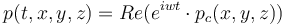where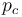is the complex-valued pressure field that you are computing,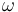is the angular frequency,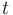is time, and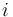is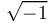.

Whenever you specify a source field in a frequency-domain simulation, you can input a complex number (a phasor) such as `pc` , where `abs(pc)` is the peak value and `real(pc)` gives you the instantaneous value at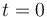This applies for any time-harmonic input. For instance, to define an acoustic pressure, an external current density, a magnetic field, or an electric potential with an RMS value of 1 and a phase angle of 30 degrees, enter the expression:

`sqrt(2)*exp(i*30[deg])`

Or, alternatively:

`sqrt(2)*(cos(30[deg])+i*sin(30[deg]))`

The following functions are useful when dealing with complex-valued numbers:

• `real(a)` returns the real component of the argument.
• `imag(a)` returns the imaginary component of the argument.
• `abs(a)` returns the magnitude of the complex-valued argument.
• `arg(a)` returns the phase.
• `conj(a)` returns the complex conjugate.
• `realdot(a,b)` returns the dot product of the two input arguments. The result is identical to `real(a*conj(b))`, but also will define the correct partial derivatives.

In many cases, especially in electromagnetics, a complex-valued vector field (such as the electric field) is of interest. So, the electric field in a 3D model would have components: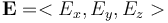For such vector fields, there will be a norm defined, in terms of the vector dotted with its complex-conjugate: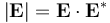When evaluating results, keep in mind that this norm is equivalent to the magnitude of the vector field, and the RMS value would be a factor of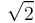less.

In electromagnetics problems, there is also usually a cycle-averaged heating computed. For example, for a frequency-domain electric currents problem, the resistive heating term is defined as: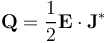### Modeling and Solving Time-Harmonic Problems

You can learn more about modeling and solving time-harmonic problems in acoustics, electromagnetics, and solid mechanics in the two-part series of blog posts on modeling the harmonic excitations of linear systems. The first part on modeling the harmonic excitations of linear systems provides an overview of concepts and conditions for which the assumption is used and outlines some options for solution approaches. The second part on modeling the harmonic excitations of nonlinear systems extends this discussion, addressing working with nonlinear systems. Both parts also connect you with models and applications where you can practice modeling several cases firsthand.

Отправьте отзыв об этой странице или свяжитесь со службой техподдержки.